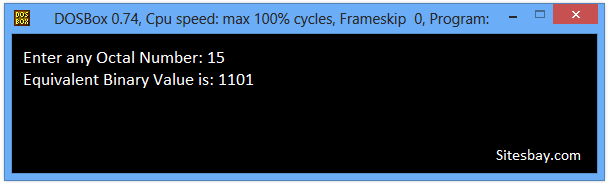# Convert Octal to Binary Program in C++

## C++ program to Convert Octal to Binary

To convert Octal number to Binary number in C++ programming, We have to ask to the user to enter the Octal number to convert it into Binary number to display the equivalent value in Binary format on screen.
In a computer system, the binary number is expressed in the binary numeral system while the octal number is in the octal numeral system. The binary number is in base 2 while the octal number is in base 8.

## Example

```Input Octal Number : 14
Euivalent Binary Number : 1100

Input Octal Number : 7
Euivalent Binary Number : 111

Input Octal Number : 10
Euivalent Binary Number : 1000
```

## C++ program to convert Octal to Binary

```#include<iostream.h>
#include<conio.h>

void main()
{
clrscr();
long int i=0;
char octnum;
cout<<"Enter any Octal Number: ";
cin>>octnum;
cout<<"Equivalent Binary Value: ";
while(octnum[i])
{
switch(octnum[i])
{
case '0' : cout<<"000";
break;
case '1' : cout<<"001";
break;
case '2' : cout<<"010";
break;
case '3' : cout<<"011";
break;
case '4' : cout<<"100";
break;
case '5' : cout<<"101";
break;
case '6' : cout<<"110";
break;
case '7' : cout<<"111";
break;
default : cout<<"\nInvalid Octal Digit "<<octnum[i];
break;
}
i++;
}
getch();
}
```

## Output

```Enter any Octal Number: 15
Equivalent Binary Value is: 1101
```## Pure VPN Privide Lowest Price VPN Just @ \$1.65. Per Month with Non Detected IP Lowest Price Non Detected IP VPN